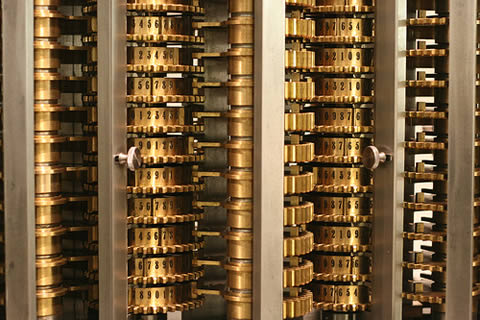Main

Filed in Encyclopedia ,Ideas ,RSubscribe to Decision Science News by Email (one email per week, easy unsubscribe)

MENTALLY MULTIPLY NUMBERS BY THEMSELVESBabbage’s Difference Engine is fueled by squares

Even in the age of ubiquitous computing, its usually faster to do a simple operation like squaring a number in your head as opposed to doing it on paper or firing up R. Everyday decision making in science needs to happen in a fast and frugal manner. Assuming you know your multiplication tables up to 10×10, here’s how to compute the squares of numbers up to 100 in your noggin (and beyond if you are willing to bootstrap).

A. If the number ends in 0, chop off the 0s, square what is left, and put back two 0s for each one you knocked off. Examples:

• 50: chopping off the zero gives 5, squaring that gives 25, replacing the 0 with two 0s gives 2500
• 100: chopping off the zero gives 1, squaring that gives 1, replacing each chopped 0 with two gives 10,000

B. If the number ends in 5, chop of the five, take what is left and multiply it by the next highest number, stick 25 on the end Examples:

• 35: chopping off the 5 gives 3, multiplying what’s left (3) by the next highest number (4) gives 12, sticking 25 on the end gives 1225
• 95: chopping off the 5 gives 9, multiplying what’s left (9) that by the next highest number (10) gives 90, sticking 25 on the end gives 9025

C. If the number is 1 greater than a number that ends in 5 or 0, first square the number ending in 5 or 0, as above, then add to this the number ending in 5 or 0 and the number. Examples:

• 51: 51 is one greater than 50, the square of which we know from above is 2500. To this we add the number ending in 5 or 0 (50) and the number (51). 2500 + 50 + 51 = 2601
• 36: 36 is one greater than 35, the square of which we know from above is 1225. To this we add the number ending in 5 or 0 (35) and the number (36). 1225 + 35 + 36 = 1296

If the number is 1 less than a number that ends in 5 or 0, first square the number ending in 5 or 0, as above, then subtract from this the number ending in 5 or 0 and the number. Examples:

• 49: 49 is one less than 50, the square of which we know from above is 2500. From this we subtract the number ending in 5 or 0 (50) and the number (49). 2500 – 50 – 49 = 2401
• 34: 34 is one less than 35, the square of which we know from above is 1225. From this we subtract the number ending in 5 or 0 (35) and the number (34). 1225 – 35 – 34 = 1156

D. If the number is 2 greater than a number that ends in 5 or 0, first square the number ending in 5 or 0, as above, then add to this four times the number that is 1 greater than the number ending in 5 or 0. Examples:

• 52: 52 is two greater than 50, the square of which we know from above is 2500. To this we add four times the number that is 1 greater than the number ending in 5 or 0 (51). 2500 + 4 * 51 = 2704
• 37: 37 is two greater than 35, the square of which we know from above is 1225. To this we add four times the number that is 1 greater than the number ending in 5 or 0 (36). 1225 + 4 * 36 = 1369

If the number is 2 less than a number that ends in 5 or 0, first square the number ending in 5 or 0, as above, then subtract from this four times the number that is 1 less than the number ending in 5 or 0. Examples:

• 48: 48 is two less than 50, the square of which we know from above is 2500. From this we subtract four times the number that is 1 less than the number ending in 5 or 0 (49). 2500 – 4 * 49 = 2304
• 33: 33 is two less than 35, the square of which we know from above is 1225. From this we subtract four times the number that is 1 less than the number ending in 5 or 0 (34). 1225 – 4 * 34 = 1089

Since every number is either 1 or 2 greater or less than a number ending in 0 or 5, we are done.

Happy squaring!

Proof of C: First part: (N+1)^2=N^2+N+(N+1), Second part (N-1)^2=N^2-N-N+1=N^2-N-(N-1)
Proof of D: First part: (N+2)^2=N^2+4N+4=N^2+4(N+1), Second part (N-2)^2=N^2-4N+4=N^2-4(N-1)

Photo credit: http://www.flickr.com/photos/mrgiles/325903759/

good by calculator

March 15, 2012 @ 2:54 am

2. How to multiply numbers in your head | Decision Science News says:

[…] the numbers are both even or both odd 1. Learn how to square numbers in your head from our earlier post 2. Take the midpoint of the two numbers and square it. For example, if the […]

March 29, 2012 @ 11:48 pm

3. mr roboto says:

i love lamp

April 28, 2012 @ 2:23 pm

### Write Comment

XHTML: <a href="" title=""> <abbr title=""> <acronym title=""> <b> <blockquote cite=""> <cite> <code> <del datetime=""> <em> <i> <q cite=""> <s> <strike> <strong>